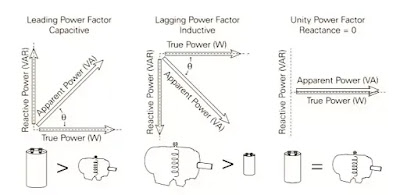# What is power factor and why is it important?

0
659

## Power Factor Definition

P.F = Cosθ

#### PF = Resistance/Impedance = R/Z## Problem with Low Power Factor

#### So In this example, a larger transformer which is capable of supplying 71.43 kVA (50/0.7) would be required. In addition to that, the size of the conductors would have to be increased to supply an increased amount of current due to low PF. So due to poor PF the cost of equipment also increased.## Types of Power Factor

#### There are three types of power factor in electrical science. These are leading power factor, Lagging power factor and Unity power factor. Let’s discuss all these types of power factor one by one in detail.